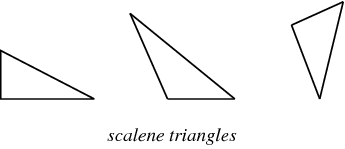C Program to Calculate Area of Scalene TrianglePosted by Samath Last Updated: January 01, 20171839

A scalene triangle is a triangle that has three unequal sides.Code:

```#include<stdio.h>
#include<math.h>

int main() {
int side1, side2, ang;
float a;

printf("\nEnter the first Side: ");
scanf("%d", &side1);

printf("\nEnter the second side: ");
scanf("%d", &side2);

printf("\nEnter the angle: ");
scanf("%d", &ang);

a = (side1 * side2 * sin((M_PI / 180) * ang)) / 2;

printf("\nArea: %f", a);
return (0);
}```